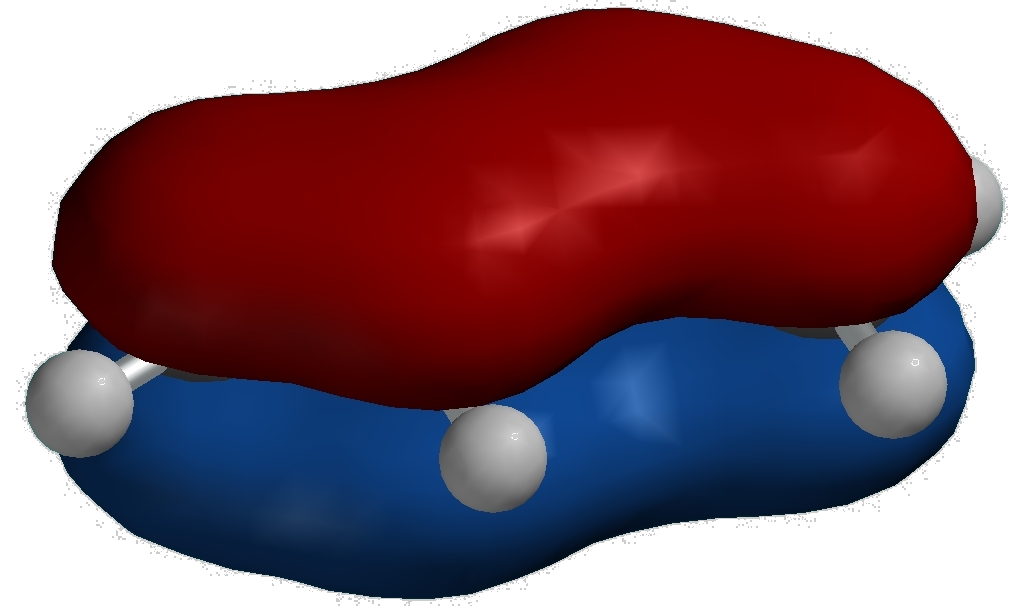Illustrated Glossary of Organic Chemistry

Orbital: A mathematical equation which defines a volume of space in which there is a specified probability of finding an electron of a specified energy. Shown below are plots of orbital equations.An s atomic orbital is spherical. It has no nodes. A p atomic orbital has two orbital lobes and a node at the nucleus. These px, py, and pz orbitals are orthogonal. The π1 molecular orbital of 1,3-butadiene has one node.